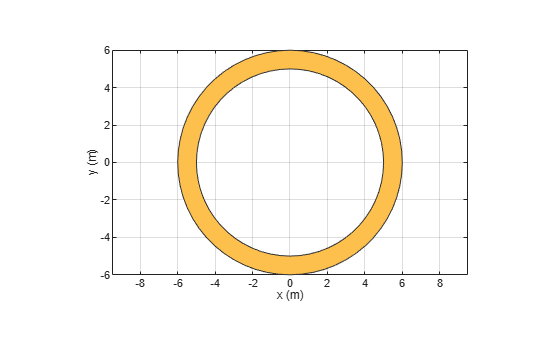# ringAnnular

Create annular ring on X-Y plane

Since R2021b

## Description

Use the `ringAnnular` object to create an annular ring on the X-Y plane.

## Creation

### Syntax

``ring = ringAnnular``
``ring = ringAnnular(Name=Value)``

### Description

example

````ring = ringAnnular` creates an annular ring on the X-Y plane.```

example

````ring = ringAnnular(Name=Value)` sets Properties using one or more name-value arguments. For example, `ringAnnular(Center=[1 1])` creates an annular ring shape centered at `[1 1]`. Properties not specified retain their default values.```

## Properties

expand all

Name of the annular ring, specified as a character vector or string scalar.

Example: `ring = ringAnnular(Name='ringannular1')`

Data Types: `char` | `string`

Center of the annular ring in Cartesian coordinates, specified as a two-element vector.

Example: `ring = ringAnnular(Center=[1 1])`

Data Types: `double`

Inner radius of the annular ring, specified as a positive scalar in meters.

Example: `ring = ringAnnular(InnerRadius=0.006)`

Data Types: `double`

Width of the annular ring, specified as a positive scalar in meters.

Example: `ring = ringAnnular(Width=3)`

Data Types: `double`

## Object Functions

 `add` Boolean unite operation on two RF PCB shapes `and` Shape1 & Shape2 for RF PCB shapes `area` Calculate area of RF PCB shape in square meters `intersect` Boolean intersection operation on two RF PCB shapes `mesh` Change and view mesh properties of metal or dielectric in PCB component `minus` Shape1 - Shape2 for RF PCB shapes `plus` Shape1 + Shape2 for RF PCB shapes `rotate` Rotate RF PCB shape about defined axis `rotateX` Rotate RF PCB shape about x-axis `rotateY` Rotate RF PCB shape about y-axis and angle `rotateZ` Rotate RF PCB shape about z-axis `subtract` Boolean subtraction operation on two RF PCB shapes `scale` Change size of RF PCB shape by fixed amount `show` Display PCB component structure or PCB shape `translate` Move RF PCB shape to new location

## Examples

collapse all

Create an annular ring shape with default properties.

`ring = ringAnnular`
```ring = ringAnnular with properties: Name: 'myringAnnular' Center: [0 0] Width: 0.0020 InnerRadius: 0.0050 ```

View the shape.

`show(ring)`Create an annular ring shape of inner radius 5 m and width 1 m.

```ring = ringAnnular(InnerRadius=5,Width=1); show(ring)```Mesh the ring at a maximum edge length of 1 m.

`mesh(ring,MaxEdgeLength=1)`## Version History

Introduced in R2021b